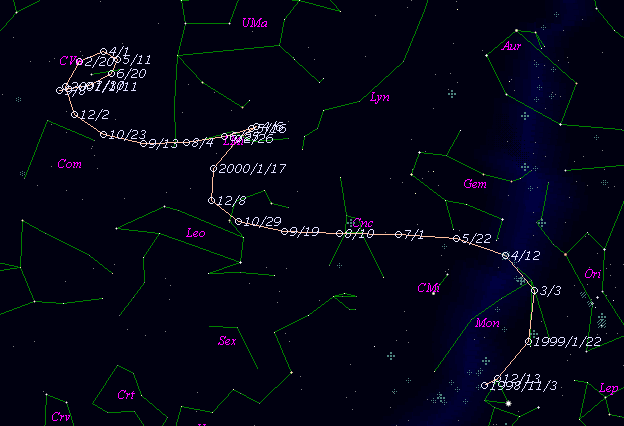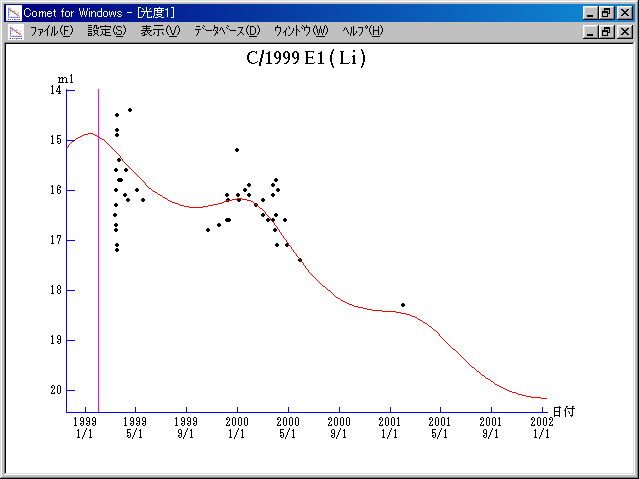# \$B%j!

C/1999 E1 ( Li )

 English version Home page Updated on May 27, 2001###\$B%W%m%U%#!<%k(B

 \$BH/8+F|(B 1999\$BG/(B 3\$B7n(B13\$BF|(B \$BH/8+8wEY(B 16.5\$BEy(B \$BH/8+ W.-d. Li (Lick Observatory)

###\$B###\$B50F;MWAG(B

```   The following improved orbital elements by Kenji Muraoka, are
from 290 observations 1999 Jan. 22 to 2001 Mar. 28, perturbations
by 9 Planets, Moon and 5 minor planets were taken into account.
The mean residual is +/- 0.66 arc seconds.

Epoch  =  1999 Jan. 22.0  TT       JDT = 2451200.5
T  =  1999 Jan. 31.70508       +/- 0.00175 (m.e.) TT
Peri. =  329.75817                +/- 0.00018
Node  =  127.83279                +/- 0.00020   (2000.0)
Incl. =   46.88668                +/- 0.00012
q  =    3.9199578              +/- 0.0001610 AU
e  =    0.7597711              +/- 0.0000068
a  =   16.3175939              +/- 0.0004884 AU
n  =    0.01495271             +/- 0.00000067
P  =   65.915                  +/- 0.0029595  years
(+/- 1.08 days)
```

###\$B@1?^(B###\$B8wEYJQ2=(B

```        m1 = 6.5 + 5 log\$B&\$(B + 10.0 log r
```##### \$B50F;MWAG\$OB<2,7r<#;a\$N7W;;\$K\$h\$k\$b\$N\$G\$9!#(B \$B@1?^\$O(B StellaNavigator Ver.2.0 for Windows (\$B%"%9%H%m%"!<%D(B \$BJTCx(B / \$B%"%9%-!<=PHG6I4)(B) \$B\$G:n@.\$7\$?\$b\$N\$G\$9!#(B \$B8wEY%0%i%U\$O(BComet for Windows\$B\$G:n@.\$7\$?\$b\$N\$G\$9!#(B